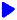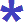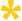Noetic Learning Math Worksheet Creator

Generate Fraction WorksheetsBack to Worksheet Creator HomeFind the Greatest Common Factor (GCF)

Number of questions:  questions per row:  Generate!Find the Least Common Multiple (LCM)

Number of questions: questions per row:   Generate!Reducing fractions to lowest terms

Number of questions: questions per row:   Generate!Compare Fractions      free sample

With denominator up to  and numerator up to

More restrictions:

Number of questions:  questions per row:  Generate!Basic operations of fractions     free sample

with denominators from     to

Use common denominators

Number of questions: questions per row:   Generate!